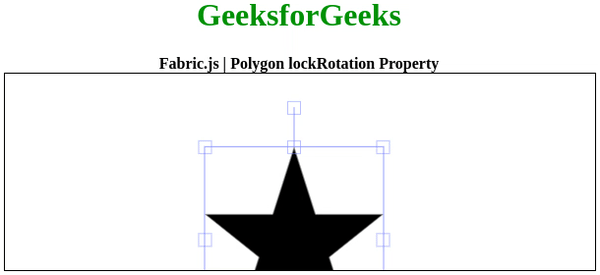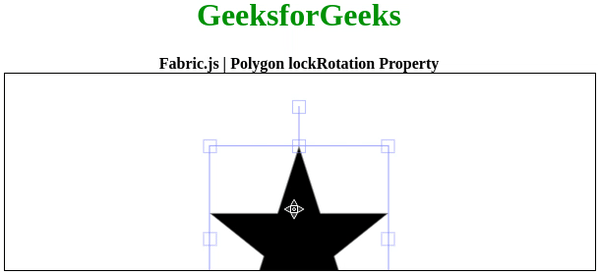# Fabric.js Polygon lockRotation Property

• Last Updated : 31 Aug, 2020

In this article, we are going to see how to lock the rotation of the canvas polygon using FabricJS. The canvas polygon means the polygon is movable and can be stretched according to requirement. Further, the polygon can be customized when it comes to initial stroke color, shape, fill color, or stroke width.

To make it possible we are going to use a JavaScript library called FabricJS. After importing the library, we will create a canvas block in the body tag that will contain the polygon. After this, we will initialize instances of Canvas and polygon provided by FabricJS and lock the rotation of the canvas polygon using lockRotation property and render the polygon on the Canvas as given in the below example.

Syntax:

```fabric.Polygon([
{ x: pixel, y: pixel },
{ x: pixel, y: pixel },
{ x: pixel, y: pixel },
{ x: pixel, y: pixel },
{ x: pixel, y: pixel }],
{
lockRotation: boolean
}
);
```

Parameters: This property accepts a single parameter as mentioned above and described below:

• lockRotation: It specifies whether to enable rotation or not.

Note: Dimension pixels is must to create a polygon.

Below examples illustrates the Fabric.JS Polygon lockRotation property in JavaScript:

Example 1: Here the locakRotation is enable.

## HTML

 ` ``<``html``> `` ` `<``head``> ``    ````    ``<``script` `src``= ``"https://cdnjs.cloudflare.com/ajax/libs/fabric.js/3.6.2/fabric.min.js"``> ``    `` `` `` ` `<``body``> ``    ``<``div` `style``=``"text-align: center;width: 600px;"``> ``        ``<``h1` `style``=``"color: green;"``> ``            ``GeeksforGeeks ``        `` ``        ``<``b``> ``            ``Fabric.js | Polygon lockRotation Property ``        `` ``    ````  ` `    ``<``canvas` `id``=``"canvas"``            ``width``=``"600"``            ``height``=``"200"``            ``style``=``"border:1px solid #000000;"``> ``    `` `` ` `    ``<``script``> `` ` `        ``// Initiate a Canvas instance ``        ``var canvas = new fabric.Canvas("canvas"); `` ` `        ``// Initiate a polygon instance ``        ``var polygon = new fabric.Polygon([ ``        ``{ x: 295, y: 10 }, ``        ``{ x: 235, y: 198 }, ``        ``{ x: 385, y: 78}, ``        ``{ x: 205, y: 78}, ``        ``{ x: 355, y: 198 }], { ``            ``lockRotation: true``        ``}); `` ` `        ``// Render the polygon in canvas ``        ``canvas.add(polygon); ``    `` `` `` ` ``

Output:Example 2: Here the locakRotation is disable.

## HTML

 ` ``<``html``> `` ` `<``head``> ``    ````    ``<``script` `src``= ``"https://cdnjs.cloudflare.com/ajax/libs/fabric.js/3.6.2/fabric.min.js"``> ``    `` `` `` ` `<``body``> ``    ``<``div` `style``=``"text-align: center;width: 600px;"``> ``        ``<``h1` `style``=``"color: green;"``> ``            ``GeeksforGeeks ``        `` ``        ``<``b``> ``            ``Fabric.js | Polygon lockRotation Property ``        `` ``    ````  ` `    ``<``canvas` `id``=``"canvas"``            ``width``=``"600"``            ``height``=``"200"``            ``style``=``"border:1px solid #000000;"``> ``    `` `` ` `    ``<``script``> `` ` `        ``// Initiate a Canvas instance ``        ``var canvas = new fabric.Canvas("canvas"); `` ` `        ``// Initiate a polygon instance ``        ``var polygon = new fabric.Polygon([ ``        ``{ x: 295, y: 10 }, ``        ``{ x: 235, y: 198 }, ``        ``{ x: 385, y: 78}, ``        ``{ x: 205, y: 78}, ``        ``{ x: 355, y: 198 }], { ``            ``lockRotation: false``        ``}); `` ` `        ``// Render the polygon in canvas ``        ``canvas.add(polygon); ``    `` `` `` ` ``

Output:My Personal Notes arrow_drop_up